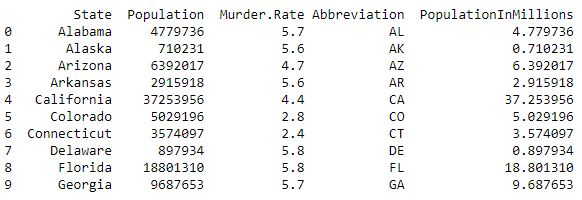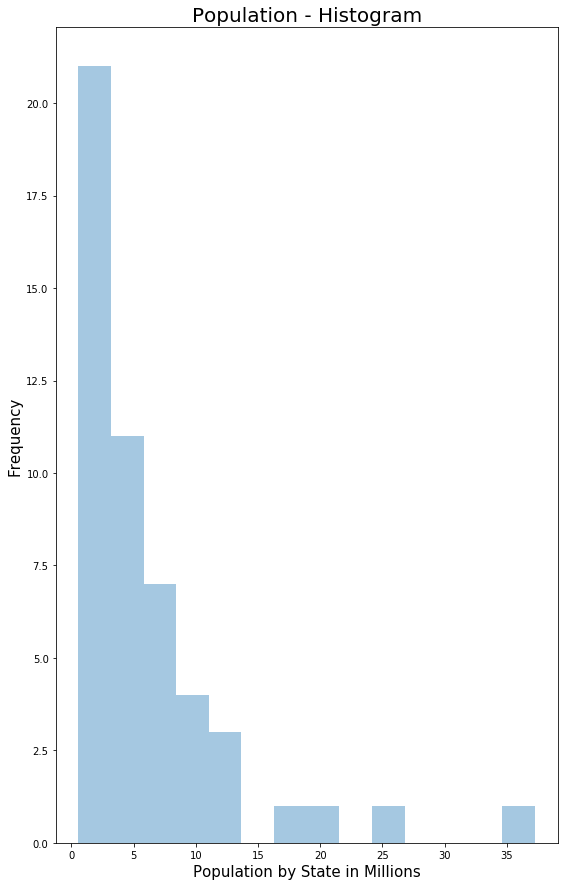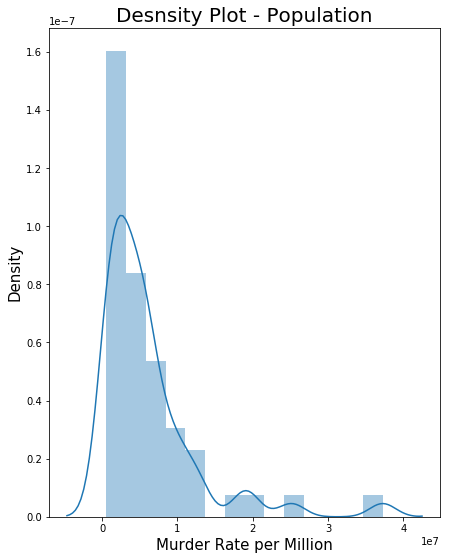# Exploring Data Distribution | Set 2

Prerequisite: Exploring Data Distribution | Set 1

Terms related to Exploration of Data Distribution

```-> Boxplot
-> Frequency Table
-> Histogram
-> Density Plot
```

To get the link to `csv `file used, click here.

 `import` `numpy as np ` `import` `pandas as pd ` `import` `seaborn as sns ` `import` `matplotlib.pyplot as plt `

 `data ``=` `pd.read_csv(``"../data/state.csv"``) ` ` `  `# Adding a new column with derived data  ` `data[``'PopulationInMillions'``] ``=` `data[``'Population'``]``/``1000000` ` `  `print` `(data.head(``10``)) `

Output :• Histogram: It is a way of visualizing data distribution through frequency table with bins on the x-axis and data count on the y-axis.

Code – Histogram

 `# Histogram Population In Millions ` ` `  `fig, ax2 ``=` `plt.subplots() ` `fig.set_size_inches(``9``,  ``15``) ` ` `  `ax2 ``=` `sns.distplot(data.PopulationInMillions, kde ``=` `False``) ` `ax2.set_ylabel(``"Frequency"``, fontsize ``=` `15``) ` `ax2.set_xlabel(``"Population by State in Millions"``, fontsize ``=` `15``) ` `ax2.set_title(``"Population - Histogram"``, fontsize ``=` `20``) `

Output :• Density Plot: It is related to histogram as it shows data-values being distributed as continuous line. It is a smoothed histogram version. The output below is the density plor superposed over histogram.

Code – Density Plot for the data

 `# Density Plot - Population ` ` `  `fig, ax3 ``=` `plt.subplots() ` `fig.set_size_inches(``7``,  ``9``) ` ` `  `ax3 ``=` `sns.distplot(data.Population, kde ``=` `True``) ` `ax3.set_ylabel(``"Density"``, fontsize ``=` `15``) ` `ax3.set_xlabel(``"Murder Rate per Million"``, fontsize ``=` `15``) ` `ax3.set_title(``"Desnsity Plot - Population"``, fontsize ``=` `20``) `

Output :My Personal Notes arrow_drop_upAspire to Inspire before I expire

If you like GeeksforGeeks and would like to contribute, you can also write an article using contribute.geeksforgeeks.org or mail your article to contribute@geeksforgeeks.org. See your article appearing on the GeeksforGeeks main page and help other Geeks.

Please Improve this article if you find anything incorrect by clicking on the "Improve Article" button below.